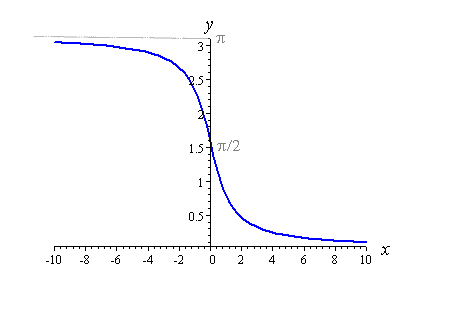# Inverse trig Inequality

• takando12

## Homework Statement

Solution set of the inequality (cot-1(x))2 -(5 cot-1(x)) +6 >0 is?

## The Attempt at a Solution

Subs cot-1(x)=y
We get a quadratic inequality in y.
y2-5y+6>0
(y-2)(y-3)>0
Using the wavy curve method, the solution set is,
y∈(-∞,2) ∪(3,∞)
So cot-1(x)<2 and cot-1(x)>3
Taking cot on both sides of the inequality,
x<cot2 and x>cot3
x∈(-∞,cot2) ∪(cot3,∞)
I'm guessing that in the step where I take cot on both sides, I'll have to change the inequality signs as arccot is a decreasing function. Is that where the problem lies?

An idea: I think you have to mind if ##\cot 2>\cot 3## or ##\cot 3>\cot 2.##

## Homework Statement

Solution set of the inequality (cot-1(x))2 -(5 cot-1(x)) +6 >0 is?

## The Attempt at a Solution

Subs cot-1(x)=y
We get a quadratic inequality in y.
y2-5y+6>0
(y-2)(y-3)>0
Using the wavy curve method, the solution set is,
y∈(-∞,2) ∪(3,∞)
So cot-1(x)<2 and cot-1(x)>3
Taking cot on both sides of the inequality,
x<cot2 and x>cot3
x∈(-∞,cot2) ∪(cot3,∞)
I'm guessing that in the step where I take cot on both sides, I'll have to change the inequality signs as arccot is a decreasing function. Is that where the problem lies?

What is the "wavy curve method"?

To clarify the answer you were given, plot the curve ##y = \text{arccos}(x)## over a broad range of ##x##, such as ##-10 \leq x \leq 10## to see what the regions ##\text{arccos}(x) < 2## and ##\text{arccos}(x) > 3## look like along the ##x##-axis.

@takando12 ,

What is the range of the arccotangent function as you are using it in your course?The plot of y=arccotan(x). In what interval of x is y≤2 or y≥3?